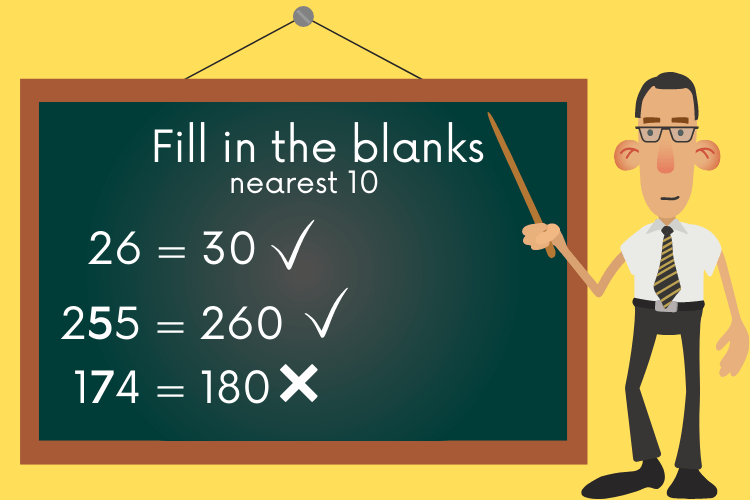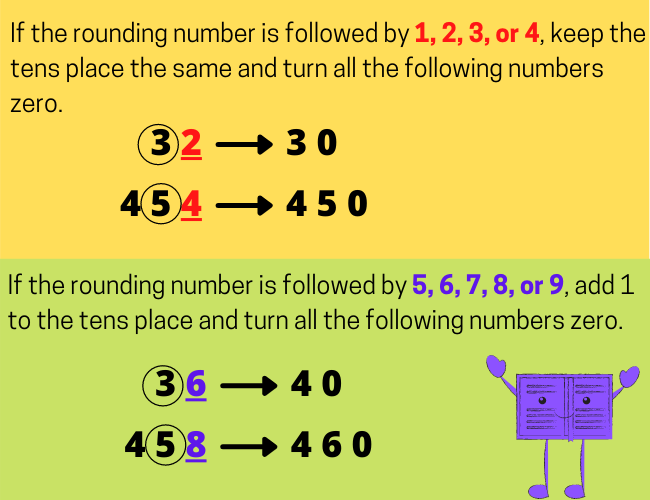# Rounding Numbers

In this article, you will learn the concept of rounding numbers, rounding numbers rules, how to round a number and more.### What is rounding?

Rounding means changing a number to make the calculation more simpler while keeping its value closer to the original number. The result is less accurate, but easier to use.

Example: 52, 58

Any guess about what the number will be after rounding?

Do you think that 52 is much more closer to 50 or to 60, is 58 much more closer to 50 or to 60?

52 is closer to 50 than 60. Therefore, 52 rounded to the nearest ten is 50.

58 is closer to 60 than 50. Therefore, 58 rounded to the nearest ten is 60.

### How to round a number:

Rule1. If the number you are rounding is followed by 1, 2, 3, or 4, keep the rounding digit the same and turn all the following numbers to zero.

Example: Round the numbers 62 and 24 to the nearest ten.

In 62, 6 is being followed by 2, so keep the tens place the same and replace the ones place by zero. Therefore we round it down to 60.

In 24, 2 is being followed by 4, so keep the tens place the same and replace the ones place by zero. Therefore we round it down to 20.

Rule2: If the number you are rounding is followed by 5, 6, 7, 8, or 9, add 1 to the number you are rounding and turn all the following numbers to zero.

Example: Round the numbers 48 and 385 to the nearest ten.

In 48, 4 is being followed by 8, so add 1 to the tens place and replace the ones place by zero. Therefore we round it up to 50.

In 385, 8 is being followed by 5, so add 1 to the tens place and replace the ones place by zero. Therefore we round it up to 390.### Rounding Numbers Free WorksheetsIf you are rounding to the nearest 10, look at the ones digit. If it is 5 or more, round it up; and if it is 4 or less, round it down.

If you are rounding to the nearest 100, look at the tens digit. If it is 5, 6, 7, 8 or 9, round it up; and if it is 1, 2, 3 or 4, round it down.

If you are rounding to the nearest 1000, look at the hundreds digit. If it is 5, 6, 7, 8 or 9, round it up; and if it is 1, 2, 3 or 4, round it down.

Try Now!

Round the following numbers to the nearest ten.

17 ________________

52 ________________

25 ________________

39 ________________

99 ________________

Round the following numbers to the nearest hundreds.

517 ________________

652 ________________

775 ________________

989 ________________

245 ________________

Translate »
Scroll to Top